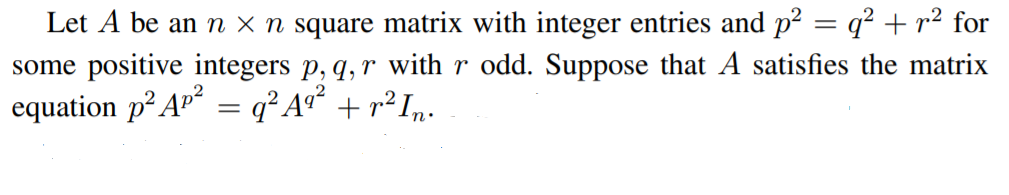# How to construct a class of matrices satisfying a given matrix equation.AnonymousHere we know that $A=I_n$ satisfies the given matrix equation. But can we find other non trivial matrix ($\neq I_n$). In other words, can we construct a class of matrices satisfying the given matrix equation.

edit retag close merge delete

If $A$ satisfies $A^{p^2} = A^{q^2} = I_n$, then that works, e.g. if $A^2=I_n$ and $p$ and $q$ are both even. If you want Sage to solve the general problem, then you can write $A$ with all symbolic entries and try to solve the resulting $n^2$ Diophantine equations. If $n$, $p$, or $q$ are large, I think this will be very difficult. What values do you have in mind?

but p and q both can not be even since r is given to be odd and they form Pythagorean triple

Yes, you're right, but the rest of my comment still applies. For example if $A^3=I$ and both $p$ and $q$ are divisible by 3. Or do you want to assume that $p$, $q$, and $r$ are relatively prime? In any case, if you imagine that $A$ has indeterminate entries and $p=5$, then you will have polynomial equations of degree 25. How would you hope to solve this?

Sort by » oldest newest most voted

The given matrix equation implies that the minimal polynomial of $A$ divides $f(x):=p^2 x^{p^2} - q^2 x^{q^2} - r^2$. It follows that $A$ can be constructed as a block diagonal matrix $\begin{bmatrix} C_g & 0\\ 0 & I_{n-d} \end{bmatrix}$ for any monic divisor $g(x)\mid f(x)$ of degree $d:=\deg g(x)\leq n$, where $C_g$ is the companion matrix of $g(x)$.

Here is a sample code that constructs and prints such matrices:

def compA(n,p,q,r):
assert p^2 == q^2 + r^2
R.<x> = PolynomialRing(ZZ)
f = p^2*x^(p^2) - q^2*x^(q^2) - r^2
for g in divisors(f):
if g.degree()>n or not g.is_monic():
continue
A = block_diagonal_matrix(companion_matrix(g), identity_matrix(n-g.degree()))
print(A)


More generally, $A$ can be taken as a block diagonal matrix with blocks $C_{g_1}, \dots, C_{g_k}$, where each $g_i(x)$ is a monic divisor of $f(x)$, and $\sum_{i=1}^k \deg g_i(x) = n$. Matrices similar to such $A$ will also satisfy the given matrix equation.

more

Thank you for your answer. But there is no output of this programme when I compile it in Sage

The code defines a function. To call it, one needs to provide values of n, p, q, r, like compA(7,5,4,3).

def compA(7,5,4,3): assert 5^2 == 4^2 + 3^2 R.<x> = PolynomialRing(ZZ) f = 5^2x^(5^2) - 4^2x^(4^2) - 3^2 for g in divisors(f): if g.degree()>7: continue A = block_diagonal_matrix(companion_matrix(g), identity_matrix(7-g.degree())) print(A)

Is it correct...but still the error message is coming

I'm not sure what error you're talking about. Click here for an example computing compA(7,15,12,9) at SageCell.

Sorry, I was making a mistake.. It is now coming very nicely. Thank you